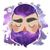# App Development

Announcements
Now Live: Qlik Sense SaaS Simplified Authoring – Analytics Creation for Everyone: READ DETAILS
cancel
Showing results for
Did you mean:Contributor II

## Color a Cell in a Table based on value from Formula

Hello, I'm struggling with the below. The formula works perfect as a Master Measure, what I want to do is highlight the cell in RED if the answer is below 120 and GREEN if equal to 120 or greater.

" IF((((((Sum({<TCODE={'IN'}>} AMOUNT))-(Sum({<TCODE={'CN'}>} AMOUNT)))-((Sum({<TCODE={'IN'}>} PROFIT))-(Sum({<TCODE={'CN'}>} PROFIT))))/(SUM(ONHAND*LTSTCOST)))*(((Sum({<TCODE={'IN'}>} PROFIT))-(Sum({<TCODE={'CN'}>} PROFIT)))/((Sum({<TCODE={'IN'}>} AMOUNT))-(Sum({<TCODE={'CN'}>} AMOUNT))))*100)>'120', 'RED',) "

Labels (3)

• ### formula

0 Replies